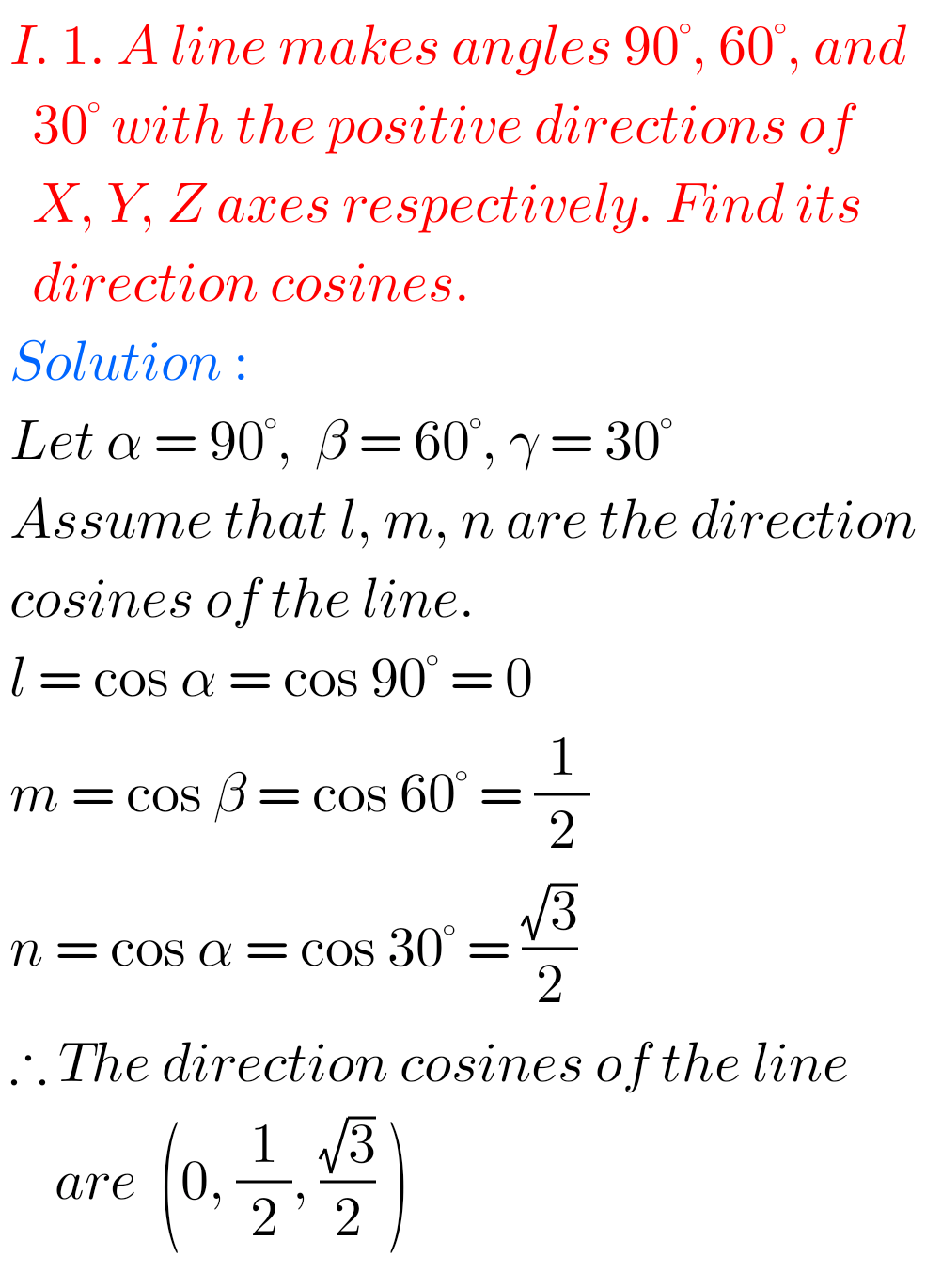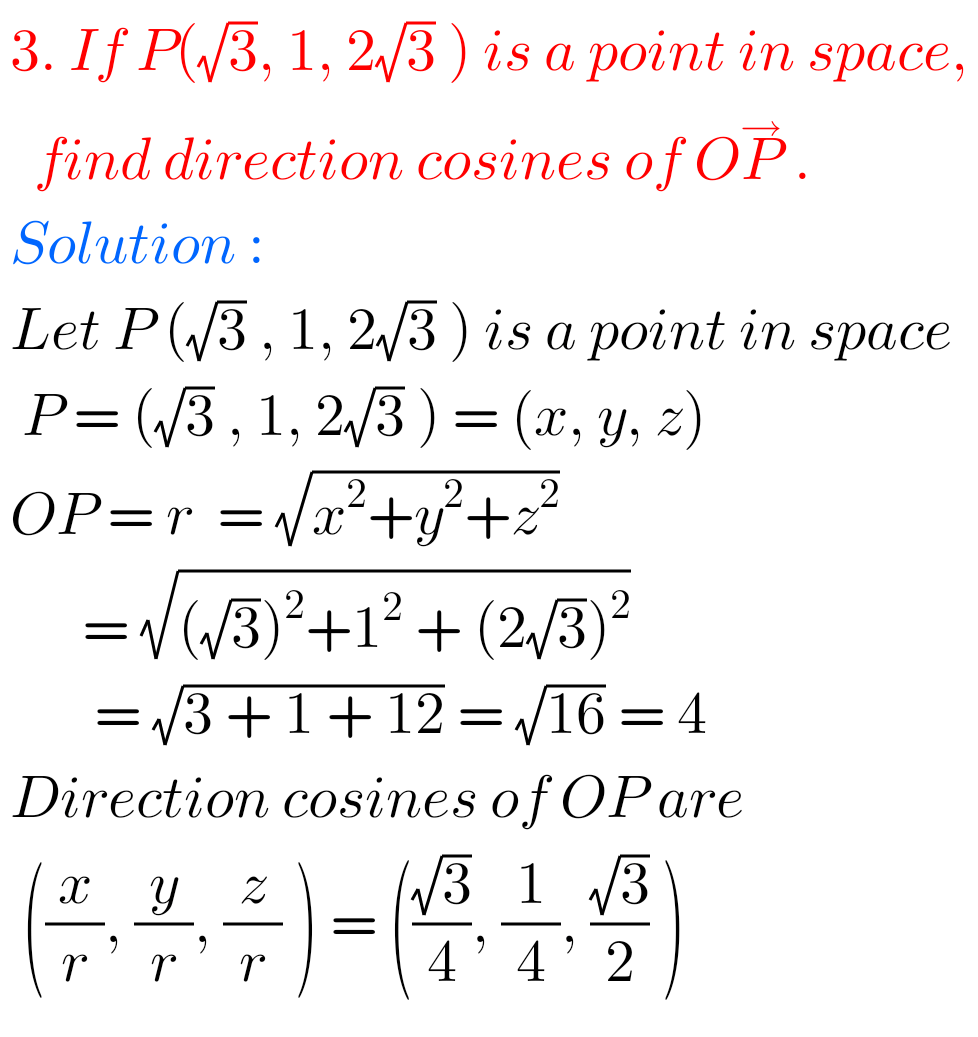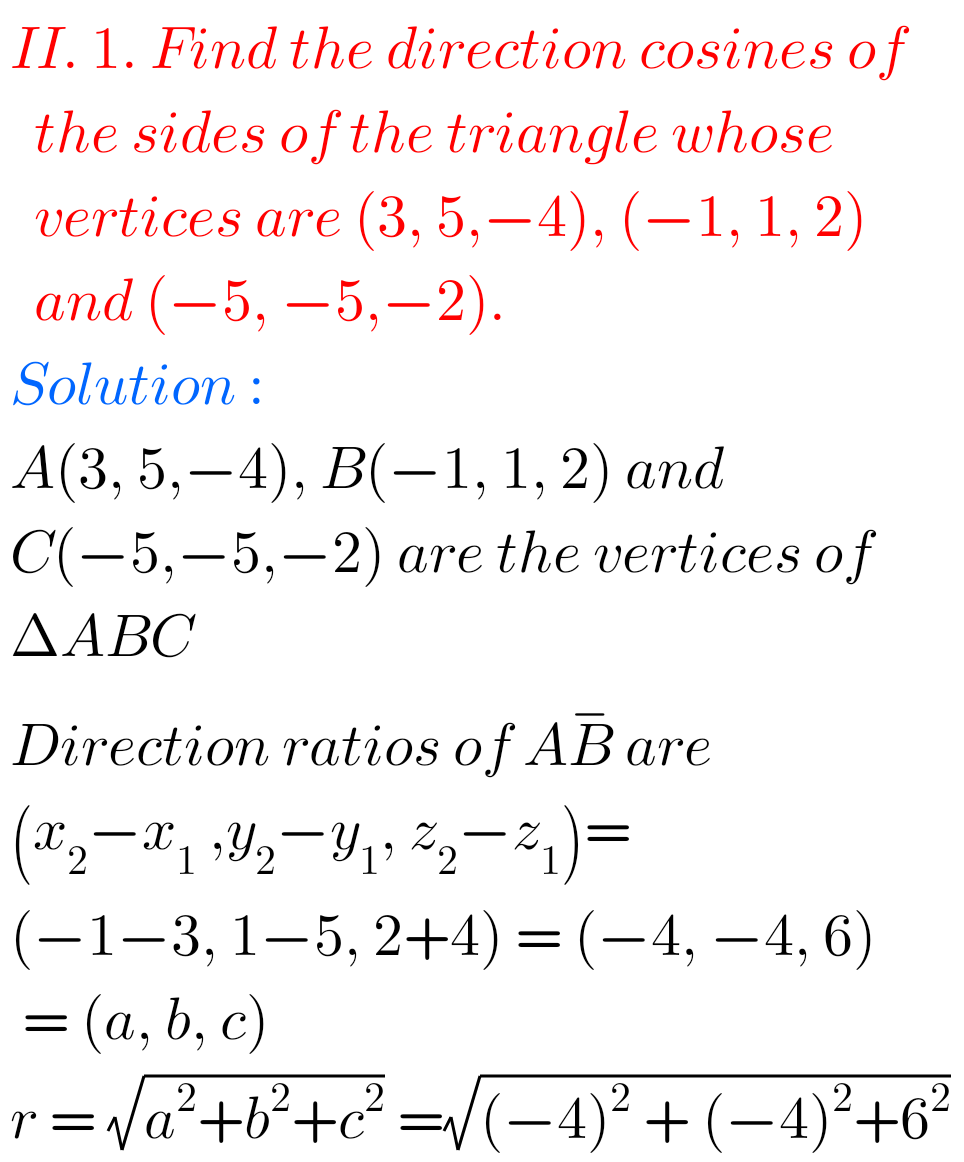# Intermediate Maths 1B Solutions for Direction Cosines and Direction Ratios Exercise 6(a)

Solutions for textbook Exercise 6(a) Direction Cosines and Direction Ratios Maths 1B Intermediate Mathematics.

Study the textbook lesson very well. Then Observe the example problems and solutions given in the textbook.

These solutions are easy to understand.

Observe the given solutions and try them in your own method.

You can also see

Inter Maths 1A textbook solutions

Inter Maths 1B textbook solutions

Inter Maths IIA textbook solutions

Inter Maths IIB textbook solutions

Dirctions cosines and direction ratios

Inter Maths Trigonometry text book solutions

M

# Direction Cosines and Direction Ratios Solutions Inter Maths 1B Exercise 6(a)

Direction Cosines and Direction Ratios

Chapter 6  – Exercise 6(a) Solutions## Maths 1B Solutions for Direction Cosines and Direction Ratios Exercise 6(a)## Solutions for Exercise 6(a) Inter Maths 1BNote : Observe the solutions and try them in your own method.

SSC Maths textbook solutions class 10

Ncert maths class 6 chapter 14 solutions

Nios maths 311 book 1 Sets 1.1 solutions

### 1 thought on “Inter Maths 1B Solutions for Direction Cosines and Direction Ratios Exercise 6(a)”

1.